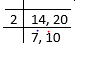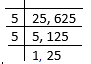# Greatest Common Divisor( HCF/GCF/GCD) Calculator

Note
• Enter the values of the two numbers whose GCF/HCF/GCD need to be calculated
• Click on the calculate button.

## What is Greatest Common Factor(GCF)

GCF stands for Greatest common factor. We sometimes called it HCF also which stands for highest commom divisor. The GCF or Highest Common Factor (HCF) of two or more given numbers is the highest of their common factors. It is also known as Greatest Common Divisor (GCD).
There are various method to find it
Method -1
Using Prime factorisation
a. Find the prime factorisation of the numbers
b. Choose the common factors in them
c. Multiply those common factors to obtain HCF
Example
Let the two number be 14 and 24
Now lets find the prime fractorisation of them
$14 = 2\times 7$
$24 = 2 \times 2 \times 2 \times 3$
Now only common factor between them is 2. So (HCF ) of 14 and 24 is 2

Method -2
Using Division algorithm
We can also find HCF using Division algorithm
Steps are
1. We place number in the line
2. We start dividing the number by least prime number which is common among all of them
3. Keep dividing till we dont find any common divisor
4. HCF is the product of the divisors
Example
Let the two number be 14 and 20So HCF of 14 and 20 is 2

Method -3
Using Factoring
a. In this we first find the factors of numbers
b. We find the common factor and then choose the highest of them
Example

Lets take the example of 40 and 64
factors of 40 are 1,2,4,8,10,20,40
factors of 64 are 1,2,4,8,16,32,64
Common factors are 1,2,4,8
So Highest common Factor is 8

Method -4
Euclid Division Lemma's
The last three methods seems good for small numbers but if we have large numbers, then factorization may take time. In that case , we may use the below technique of Euclid Division lemma's
We know that for any two integers a,b. we can write following expression
$a=bq + r \; , \; 0 \leq r < b$
If r=0 ,then
HCF( a,b) =b
If r >0 , then
HCF (a,b) = HCF ( b,r)
Again expressing the integer b,r in Euclid's Division Lemma, we get
$b=pr + r_1$
HCF ( b,r)=HCF ( r,r1)
Similarly successive Euclid 's division can be written until we get the remainder zero, the divisor at that point is called the HCF of the a and b
Example
Lets find the HCF of 867 and 255 using Euclid division algorithm
$867=255 \times 3 + 102$
Now HCF(867,255)=HCF(255,102)
Again writing 255,102 is Euclid division formula
$255=102 \times 2 + 51$
Now HCF(255,102)=HCF(102,51)
Again writing 102,51 is Euclid division formula
$102=51 \times 2 + 0$
Now r =0, 51 is HCF (102,51)
51 is HCF ( 867,255).

The Method 1,2,3 can be used for more than 2 numbers at the same time. But for method 4, we need to find the HCF of first two numbers and then whatever number we find from them has to again used to other number to find the HCF
HCF (a, b,c) = HCF ( HCF(a,b) , c)

Example of Few questions where you can use this GCF Calculator
Question 1
Find the GCF of 25 and 625 .
Solution
Using Prime Factorisation
$25 = 5 \times 5$
$625 = 5 \times 5 \times \times 5 \times 5$
HCF = $5 \times 5=25$
Using Division AlgorithmSo ,HCF = $5 \times 5=25$
Using Factoring
Factors of 25 are 1, 5,25
Factors of 625 are 1, 5,25, 125, 625
Highest Common factor is 25
Using Euclid Divison lemma
$a=bq + r \; , \; 0 \leq r < b$
$625 = 25 \times 25 + 0$
So HCF is 25

Question 2
Find the GCF of 616 and 32.
Solution
Using Euclid Divisiom Lemma
$a=bq + r \; , \; 0 \leq r < b$
$616 =32 \times 19 + 8$
Now HCF( 616,32) = HCF ( 32,8)
Again applying euclid theorem
$32 = 8 \times 4 + 0$
Now r=4, So HCF( 32,8) = 8
Therefore HCF( 616,32) = HCF ( 32,8) =8

Question 3
Find the GCF of 16 ,64 , 512
Solution
Now HCF (16 ,64 , 512) = HCF ( HCF(16,64) ,512)
Lets find HCF(16,64)
Using euclid Lemma
$64 = 16 \times 4 + 0$
Therefore HCF(16,64) =16
So, HCF (16 ,64 , 512) = HCF ( HCF(16,64) ,512)= HCF ( 16, 512)
Lets find HCF(16,512)
Using euclid Lemma
$512 = 16 \times 32 + 0$
Hence HCF ( 16, 512)= 16
Therefore
HCF (16 ,64 , 512) = HCF ( HCF(16,64) ,512)= HCF ( 16, 512)= 16

Question 4
Find the GCF of 88 and 121.
Solution
Using Euclid Divisiom Lemma
$a=bq + r \; , \; 0 \leq r < b$
$121 = 88 \times 1 + 33$
Now HCF( 121,88) = HCF (88,33)
Again applying Euclid Lemma
$88= 33 \times 2 + 22$
Now HCF( 121,88) = HCF (88,33)= HCF( 33,22)
$33 = 22 \times 1 + 11$
Now HCF( 121,88) = HCF (88,33)= HCF( 33,22)= HCF(22,11)
$22 = 11 \times 2 + 0$
So HCF (22,11) =11
Therefore
HCF( 121,88) = HCF (88,33)= HCF( 33,22)= HCF(22,11)=11
Now HCF( 121,88) = HCF (88,33)= HCF( 33,22)= HCF(22,11)

Practice questions
• Find the GCF of 65 and 117.
• Find the HCF of 135 and 90.

## How GCF calculator works

This calculator find the HCF using Euclid Division Lemma's
For a and b any two positive integer, we can always find unique integer q and r such that
$a=bq + r \; , \; 0 \leq r < b$
It is basic concept and it is restatement of division
a is called dividend
b is called divisor
q is called quotient
r is called remainder.
If r =0, then b is divisor of a.
If r >0 , then
HCF (a,b) = HCF ( b,r)
Again expressing the integer b,r in Euclid's Division Lemma, we get
$b=pr + r_1$
HCF ( b,r)=HCF ( r,r1)
Similarly successive Euclid 's division can be written until we get the remainder zero, the divisor at that point is called the HCF of the a and b